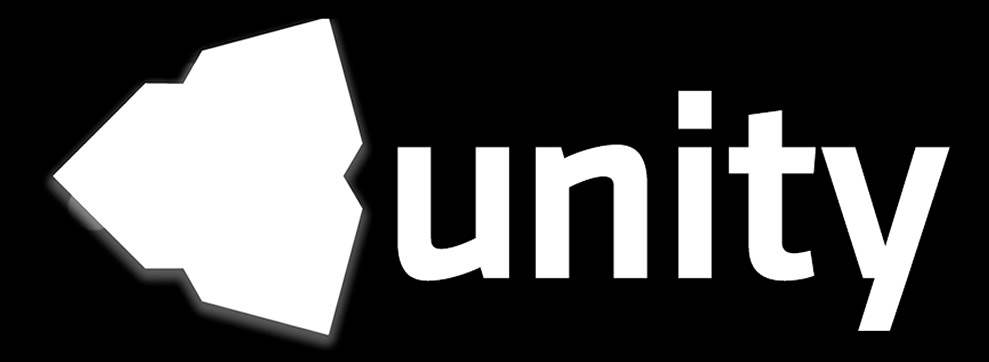# Jim's GameDev Blog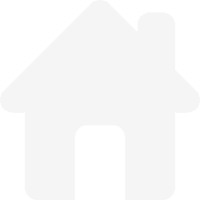# Billboard Reflection

2016-9-7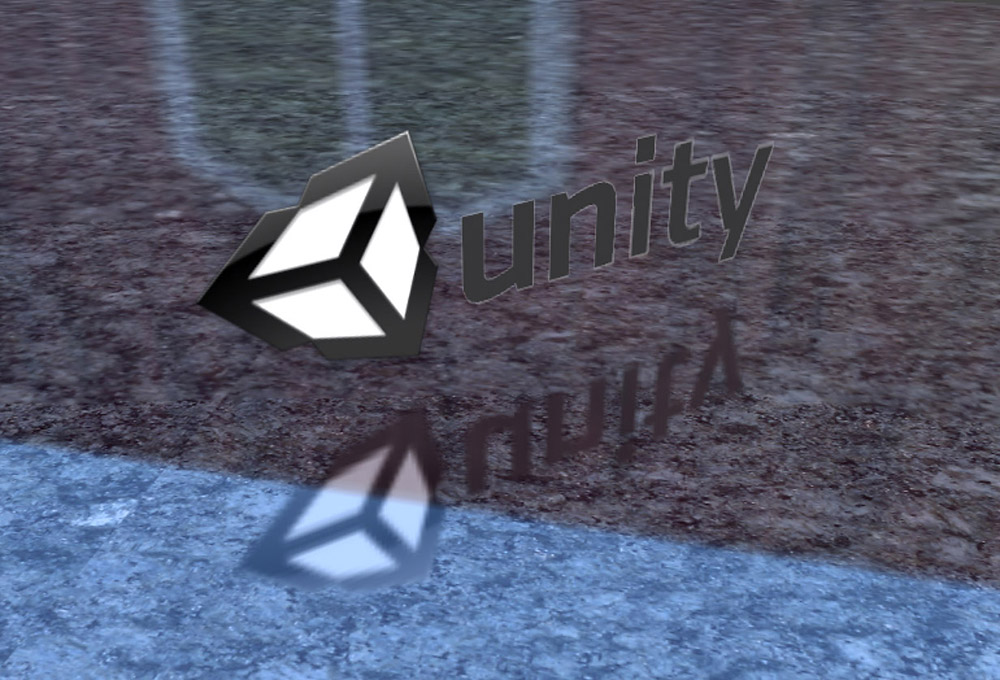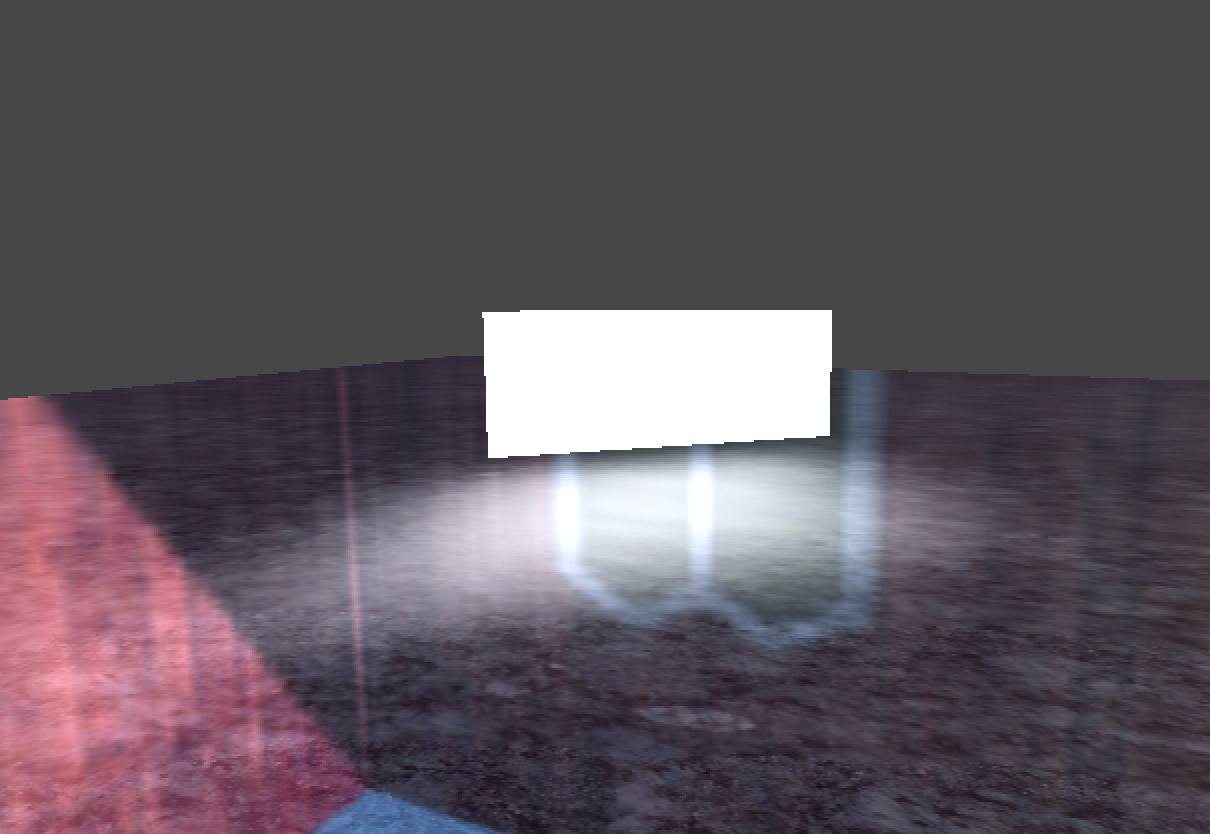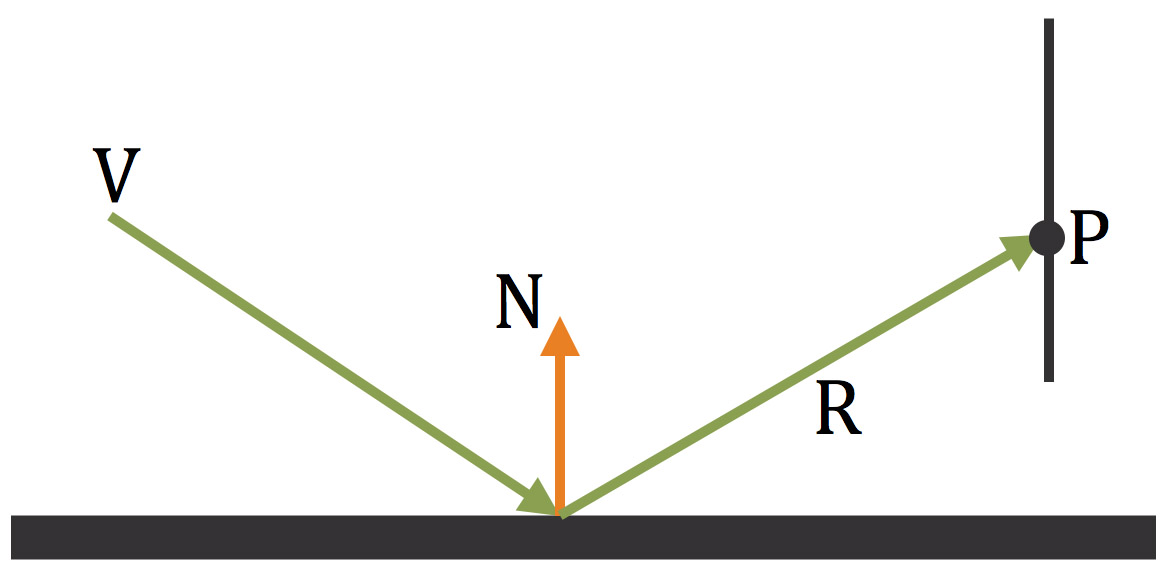V : 视线向量

N : 法线向量

R : 反射向量

P : 反射向量于面片的交点

$(P_{x}-P_{o}) \cdot P_{N} = 0$

$P_{x}$ : 平面上任意一点

$P_{o}$ : 平面上另一个点

$P_{N}$ : 平面的法向量

$R(t) = R_{o} + R_{D} * t$

$R_{o}$ : 射线的起点

$R_{D}$ : 射线的方向

$t$ : 射线在方向上经过的距离

\begin{align} (R_{o} + R_{D} * t - P_{o}) \cdot P_{N} &= 0 \\ { (P_{o} - R_{o}) \cdot P_{N} \over R_{D} \cdot P_{N} } &= t \end{align}

// Application code
material.SetVector("_PlanePos", plane.position);
material.SetVector("_PlaneN", plane.forward);
material.SetMatrix("_PlaneW2O", plane.worldToLocalMatrix);


// Shader code
// fragment
float3 viewDir = normalize(IN.worldPos - _WorldSpaceCameraPos);
float3 worldNormal = normalize(IN.worldNormal);
float3 reflectDir = normalize(reflect(viewDir, worldNormal));

float3 Po_Ro = _PlanePos - IN.worldPos;
float dot_RD_PN = dot(reflectDir, _PlaneN);
float t = dot(Po_Ro, _PlaneN) / dot_RD_PN;
// 这里得到的 intersectionPos 就是射线和平面的交点
float3 intersectionPos = IN.worldPos + reflectDir * t;
// 由于这个交点是在世界空间坐标下的，所以将其转换到平面的模型坐标系下
intersectionPos = mul(_PlaneW2O, float4(intersectionPos,1));
// 将模型坐标系下的坐标映射为 uv 坐标
// 由于我使用的是一个单位长度为 1 的 Plane
// 所以是将模型坐标系下的 -0.5~0.5 映射为 0~1
intersectionPos += 0.5;
float2 uv = intersPos.xy;
fixed4 reflCol = 0;
// 超出 0~1 纹理坐标范围外的区域要忽略掉
if (intersPos.x < 0 || intersPos.x > 1 || intersPos.y < 0 || intersPos.y > 1)
{
reflCol = 0;
}
else
{
reflCol = tex2D(_ReflTex, float2(1-uv.x, uv.y));
}
// 混合到固有色中
o.Albedo = o.Albedo * (1 - reflcol.a) + reflcol.rgb * reflcol.a;